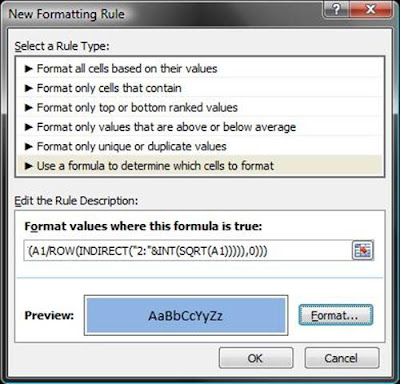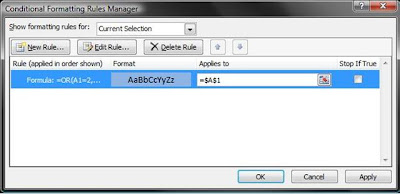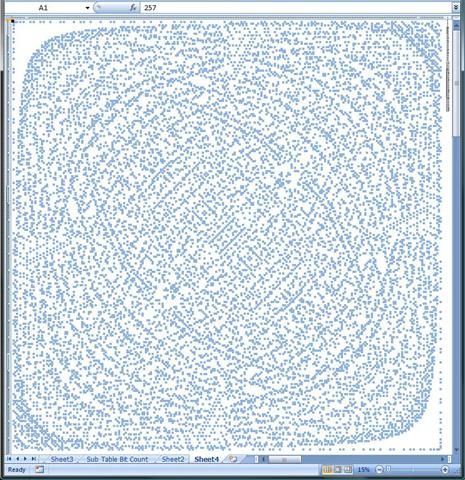## Links to stuff on this blog

Use the Site Index of Projects page link above for an easier way to find stuff on my blog that you might be looking for!

## Sunday, July 10, 2011

### Conditional Formatting in Excel and Prime Numbers

Numbers are cool and fun to play with in my opinion, especially Prime Numbers. I wrote about this some time ago in a post HERE and I've had some ideas since then. In that post I was using a Excel spread sheet with some Visual Basic code to create a number sequence then I was using another function to check if a number in a particular cell was prime. The function was scanning through a list of prime numbers in the spreadsheet and if it found a match the formatting for the cell that matches was changed to blue using Copy Formats in Excel 2007 instead of the Conditional Formatting idea. To do all that in Visual Basic for Excel was fun but overly complicated as pointed out to me by Codemann8. He commented on that last post and left a formula for Excel that can check to see if a number is prime! It's a cryptic and really cool formula that he didn't take credit for (it's out on the web) but it will check for prime numbers. I finally got around to trying this formula out and I used it to highlight prime numbers in a simple multiplication chart that uses modulo arithmetic. Below is what the Multiplication Modulo Chart looked like but I also want to show how to set this formula up to control Conditional Formatting in Excel 2007 because it took me awhile to get it to work.Excel 2007 Conditional Formatting in Modulo Multiplication Table

The above picture shows what the spreadsheet ended up looking like. Along the top and down the left side are the numbers 1-257 (only 1-23 are shown) and in the middle are the products of those numbers - a multiplication chart. In the upper left corner in the highlighted cell is the modulus of multiplication. In other words the numbers in the top and left are being multiplied modulo 23 in this case. The blue cells are the prime numbers being highlighted by the formula below using the Conditional Formatting function. At the end of this post I made a short video that cycles the multiplication chart through various number sequences showing the various patterns the prime numbers make! Really Cool!!!

Below is the formula that Codemann8 left in a comment on another post I wrote and it checks to see if a number is prime returning the Excel TRUE condition if it is. If you 'Google' that formula you will get a bunch of pages that talk about it. Whoever came up with this formula had their thinking cap on!
=OR(A1=2,A1=3,ISNA(MATCH(TRUE,A1/ROW(INDIRECT("2:"&INT(SQRT(A1))))=INT(A1/ROW(INDIRECT("2:"&INT(SQRT(A1))))),0)))
Click below to read more about how to set up Conditional Formatting in Excel 2007 to use this formula and to see the boring exciting video I made of the spreadsheet cycling through various number sequences, highlighting the prime numbers as it goes!

I'm not even going to attempt to explain how that formula does what it does. There is info on the web describing how it works it's magic if you are interested. One thing I will mention is that this formula has to be entered as an Array Function. In other words to get it to work you have to put it in cell A1 and type CTRL+SHIFT+ENTER, don't just type in ENTER after putting it in the spreadsheet. The CTRL+SHIFT+ENTER keystroke will put {} around the formula and tell Excel that you know what you are doing and treat this formula differently than if you had just entered ENTER. Search the web for Excel Array Functions if you want to know more about it.

I'm going to go through the steps to use the formula because it took me awhile to get it to work so I thought that other people might have the same problem. First off I copied the formula into a cell on the spreadsheet and clicked ENTER. Then i went back to that cell, placed my cursor in the formula bar someplace in the formula and performed the CTRL+SHIFT+ENTER trick to turn it into an Array Function. Then I opened the Conditional Formatting menu as shown below.Conditional Formatting Excel 2007
At the bottom of the Conditional Formatting menu there is a Manage Rules option, that is what you want to select. When you select that the Conditional Formatting Rules Manager dialog opens like below. Click on the image above and look close at the little paint brush icon in the upper left corner in the Clipboard area (where Paste is). That is the Formatting Paintbrush and it will be important later.Conditional Formatting Rules Manager Excel 2007
In the Conditional Formatting Rules Manager click on the New Rule box in the upper left. We are going to be adding a new rule! A dialog box like below will open for adding a New Formatting Rule.New Formatting Rule Excel 2007 Dialog Box (Note the formula from above and the Preview: blue formatting)
In the dialog box "Format values where this formula it true" paste the array formula that was in the cell. When I put it in the box as shown above the {} that were around the formula disappeared. I don't know why that happens but just pasting in the formula didn't work for me. I had to first paste the formula into an cell in the spreadsheet and use the CTRL+SHIFT+ENTER step to make it an array function then copy and past it into the box as shown above.

After putting the formula in the box I set the Format to Blue using the Format button that it highlighted above. When I hit the OK button the Conditional Formatting Rules Manager dialog opened again but now it had the formula and the formatting in it as shown below.Conditional Formatting Rules Manager with a formula in it

I clicked Apply to exit the above dialog box and get back to the spreadsheet. At that point I used the Format Paintbrush that I mentioned above to apply this formatting rule to the entire spreadsheet. The Formatting Paintbrush works like a copy and past, pretty easy.

As I mentioned above the spreadsheet that I made is a big multiplication table that does the multiplication modulo a number in cell A1. With the formatting rule that I mentioned above the numbers in the spreadsheet that are prime are highlighted in blue. The picture below is a screen shot of the spreadsheet multiplying modulo 257 - doesn't it look neat?Excel 2007 Conditional Formatting in Modulo Multiplication Table (MOD 257)
I'm wondering if this would make a good quilt pattern or maybe a tablecloth? Not sure but the really neat thing is that I wrote a couple of Visual Basic scripts that cycle the multiplication modulo all prime numbers less than 257 and also every third number starting with 3 then starting with 4. The highlighted prime numbers make neat patterns and in the case of the prime numbers it appears to zoom into a speckled square like what is shown above. have a look at the below video and if you have any questions or comments please contact me with an email at ottobelden@yahoo.com or by leaving a comment below. Enjoy the video!!

Check out other MC Excel stuff I have written about in the Site Index of Projects link at the top of the blog.